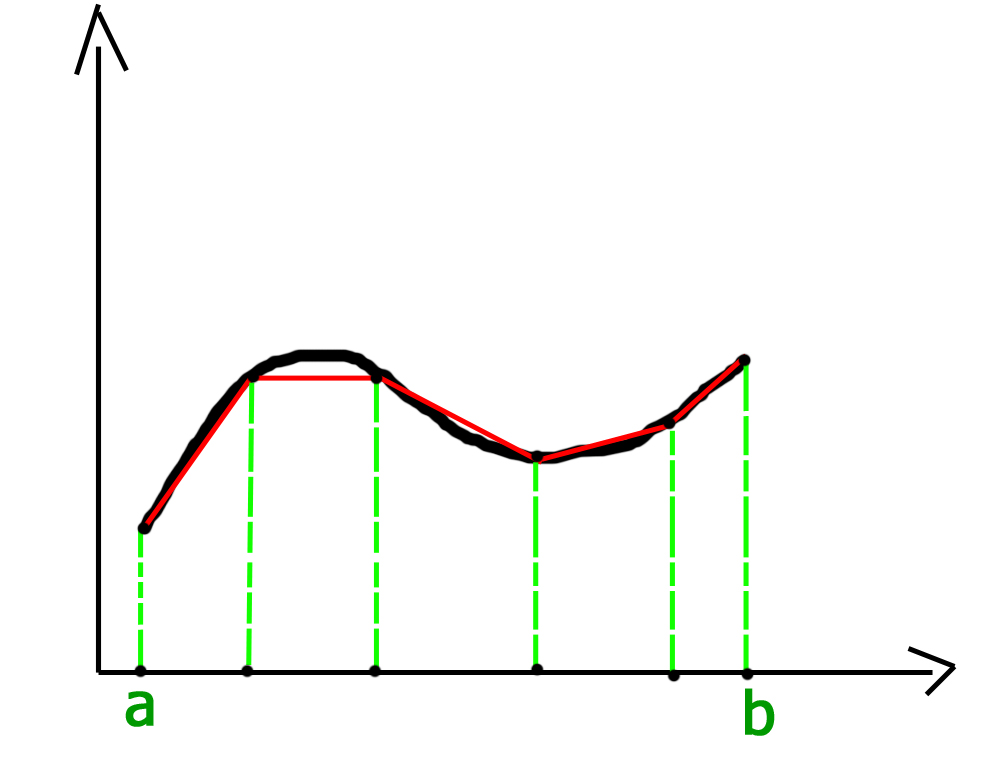Trapezoidal Rule for Approximate Value of Definite Integral

• Difficulty Level : Easy
• Last Updated : 17 Jun, 2021

In the field of numerical analysis, Trapezoidal rule is used to find the approximation of a definite integral. The basic idea in Trapezoidal rule is to assume the region under the graph of the given function to be a trapezoid and calculate its area.
It follows that:For more accurate results the domain of the graph is divided into n segments of equal size as shown below:Grid spacing or segment size h = (b-a) / n.
Therefore, approximate value of the integral can be given by:C++

 // C++ program to implement Trapezoidal rule#include // A sample function whose definite integral's// approximate value is computed using Trapezoidal// rulefloat y(float x){    // Declaring the function f(x) = 1/(1+x*x)    return 1/(1+x*x);} // Function to evaluate the value of integralfloat trapezoidal(float a, float b, float n){    // Grid spacing    float h = (b-a)/n;     // Computing sum of first and last terms    // in above formula    float s = y(a)+y(b);     // Adding middle terms in above formula    for (int i = 1; i < n; i++)        s += 2*y(a+i*h);     // h/2 indicates (b-a)/2n. Multiplying h/2    // with s.    return (h/2)*s;} // Driver program to test above functionint main(){    // Range of definite integral    float x0 = 0;    float xn = 1;     // Number of grids. Higher value means    // more accuracy    int n = 6;     printf("Value of integral is %6.4f\n",                  trapezoidal(x0, xn, n));    return 0;}

Java

 // Java program to implement Trapezoidal rule class GFG{    // A sample function whose definite    // integral's approximate value     // is computed using Trapezoidal    // rule    static float y(float x)    {        // Declaring the function        // f(x) = 1/(1+x*x)        return 1 / (1 + x * x);    }         // Function to evaluate the value of integral    static float trapezoidal(float a, float b, float n)    {        // Grid spacing        float h = (b - a) / n;             // Computing sum of first and last terms        // in above formula        float s = y(a) + y(b);             // Adding middle terms in above formula        for (int i = 1; i < n; i++)            s += 2 * y( a + i * h);             // h/2 indicates (b-a)/2n. Multiplying h/2        // with s.        return (h / 2) * s;    }         // Driver code    public static void main (String[] args)    {        // Range of definite integral        float x0 = 0;        float xn = 1;             // Number of grids. Higher        // value means more accuracy        int n = 6;             System.out.println("Value of integral is "+                           Math.round(trapezoidal(x0, xn, n)                           * 10000.0) / 10000.0);    }} // This code is contributed by Anant Agarwal.

Python3

 # Python3 code to implement Trapezoidal rule # A sample function whose definite# integral's approximate value is# computed using Trapezoidal ruledef y( x ):         # Declaring the function    # f(x) = 1/(1+x*x)    return (1 / (1 + x * x))     # Function to evaluate the value of integraldef trapezoidal (a, b, n):         # Grid spacing    h = (b - a) / n         # Computing sum of first and last terms    # in above formula    s = (y(a) + y(b))     # Adding middle terms in above formula    i = 1    while i < n:        s += 2 * y(a + i * h)        i += 1             # h/2 indicates (b-a)/2n.    # Multiplying h/2 with s.    return ((h / 2) * s) # Driver code to test above function# Range of definite integralx0 = 0xn = 1 # Number of grids. Higher value means# more accuracyn = 6print ("Value of integral is ",     "%.4f"%trapezoidal(x0, xn, n))  # This code is contributed by "Sharad_Bhardwaj".

C#

 // C# program to implement Trapezoidal// rule.using System; class GFG {         // A sample function whose definite    // integral's approximate value    // is computed using Trapezoidal    // rule    static float y(float x)    {                 // Declaring the function        // f(x) = 1/(1+x*x)        return 1 / (1 + x * x);    }         // Function to evaluate the value    // of integral    static float trapezoidal(float a,                       float b, float n)    {                 // Grid spacing        float h = (b - a) / n;             // Computing sum of first and        // last terms in above formula        float s = y(a) + y(b);             // Adding middle terms in above        // formula        for (int i = 1; i < n; i++)            s += 2 * y( a + i * h);             // h/2 indicates (b-a)/2n.        // Multiplying h/2 with s.        return (h / 2) * s;    }         // Driver code    public static void Main ()    {                 // Range of definite integral        float x0 = 0;        float xn = 1;             // Number of grids. Higher        // value means more accuracy        int n = 6;             Console.Write("Value of integral is "          + Math.Round(trapezoidal(x0, xn, n)                        * 10000.0) / 10000.0);    }} // This code is contributed by nitin mittal.



Javascript



Output:

Value of integral is 0.7842

This article is contributed by Harsh Agarwal. If you like GeeksforGeeks and would like to contribute, you can also write an article using write.geeksforgeeks.org or mail your article to review-team@geeksforgeeks.org. See your article appearing on the GeeksforGeeks main page and help other Geeks.